基于 HTML5 WebGL 构建智能数字化城市 3D 全景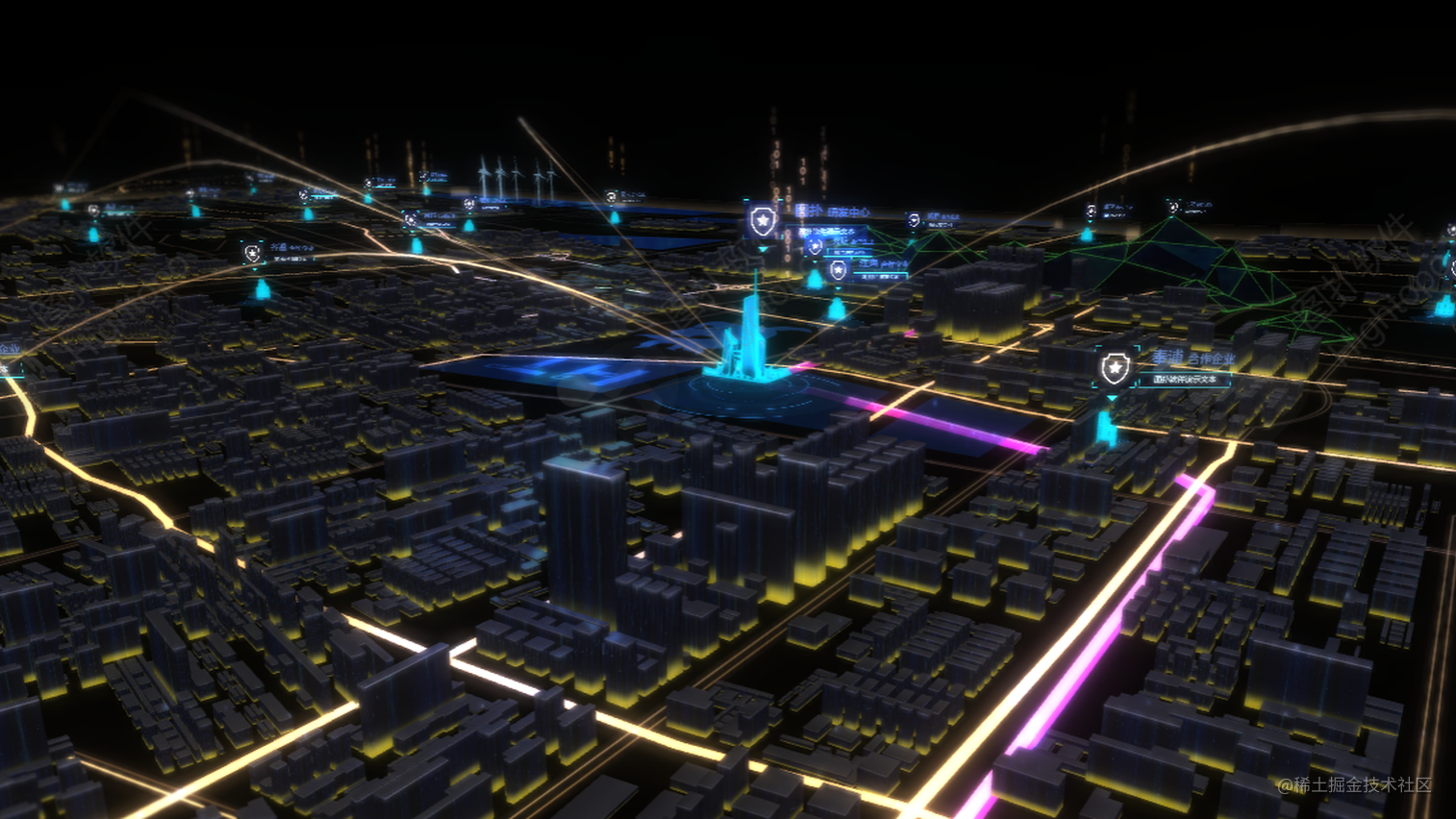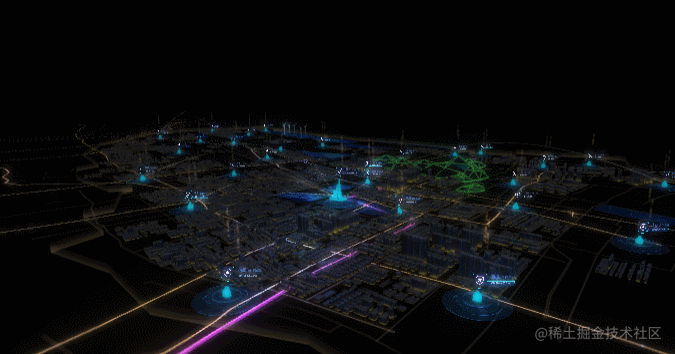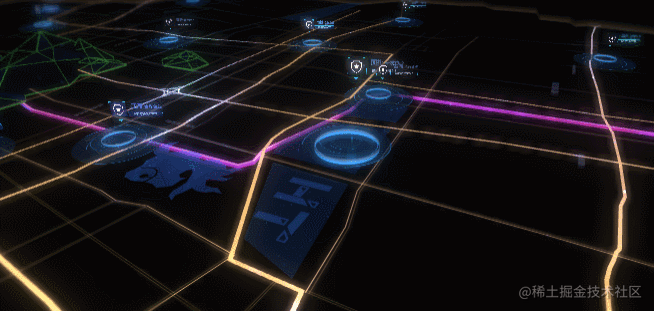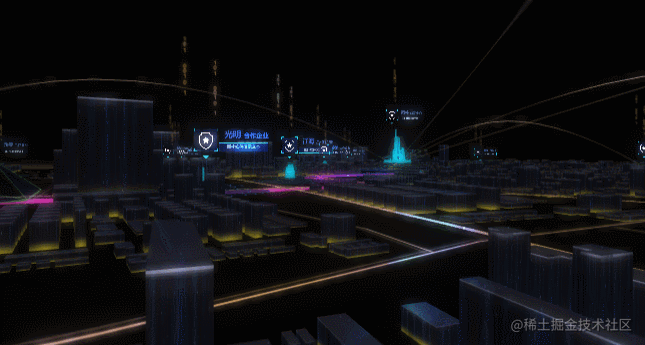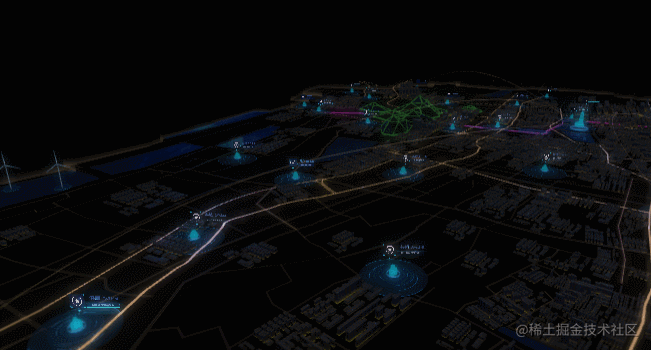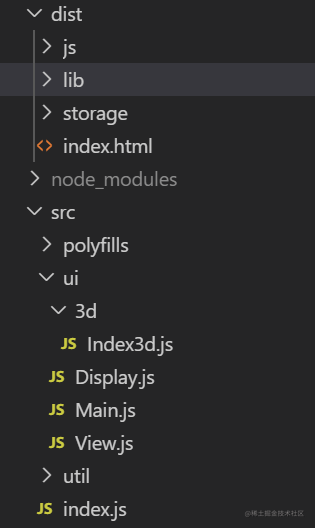storage 保存的便是 3D 场景文件。 index.js 是 src 下的入口文件，创建了一个 由 main.js 中导出的 Main 类，Main 类创建了一个 3D 画布，用于绘制我们的 3D 场景，如下

import event from '../util/NotifierManager';
import Index3d from './3d/Index3d';
import { INDEX, EVENT_SWITCH_VIEW } from '../util/constant';

export default class Main {
constructor() {
let g3d = this.g3d = new ht.graph.Graph3dView(),

//将3d图纸添加到dom对象中

this.event = event;
//创建一个Index3d类，作为场景初始化
this.index3d = new Index3d(g3d);
//调用switch方法派发EVENT_SWITCH_VIEW事件，并传入事件类型 INDEX
this.switch(INDEX);
}
switch(key = INDEX) {
event.fire(EVENT_SWITCH_VIEW, key);
}
//
}复制代码

class NotifierManager {
constructor() {
this._eventMap ={};
}

add(key, func, score, first = false) {
let notify = this._eventMap[key];
if (!notify) notify = this._eventMap[key] = new ht.Notifier();

}

remove(key, func, score) {
const notify = this._eventMap[key];
if (!notify) return;

notify.remove(func, score);
}

fire(key, e) {
const notify = this._eventMap[key];
if (!notify) return;

notify.fire(e);
}
}

const event = new NotifierManager();
export default event;复制代码

notify.fire() 和 notify.add() 分别是派发和订阅事件，类似于设计模式中的订阅者模式，我们很清楚的能看到，NotifierManager 类就是对 HT 原有的派发器做了一个简单地封装 ，并在创建 main 对象的时候，调用event.fire() 自动派发了 EVENT_SWITCH_VIEW 这一事件并且传入了事件类型 Index 。

import event from "../util/NotifierManager";
import util from '../util/util';
import { EVENT_SWITCH_VIEW } from "../util/constant";

export default class View {
constructor(view) {
this.url = '';
this.key = '';
this.active = false;
this.view = view;
this.dm = view.dm();

this.handleSwitch(key);
});
}
handleSwitch(key) {
if (key === this.key) {
if (!this.active) {
this.active = true;
this.onUp();
}
this.dm.clear();
util.deserialize(this.view, this.url, this.onPostDeserialize.bind(this));
}
// 目前是这个场景，执行 tearDown
else if (this.active) {
this.onDown();
this.active = false;
}
}
/**
* 加载这个场景前调用
*/
onUp() {
}
/**
* 离开这个场景时会调用
*/
onDown() {
}
/**
* 加载完场景处理
*/
onPostDeserialize() {
console.log(this)
}
}复制代码

deserialize: (function() {
let cacheMap = {};
/**
* 加载 json 并反序列化
*
*/
return function(view, url, cb, notUseCache) {
let json, cache = !notUseCache;
if (!notUseCache) {
json = cacheMap[url];
}
else {
cache = false;
}
// 不使用缓存，重新加载
view.deserialize(json || url, (json, dm, view, list) => {
cacheMap[url] = json;
cb && cb(json, dm, view, list, cache);
}
})()复制代码

moveEyeAction: function(g3d,moveEyeConfig,cover){
if (!moveEyeConfig) return;
let moveEye = function(obj,time,eas = 'liner'){
return new Promise((res,rej) => {
g3d.setEye(obj.initEye);
g3d.setCenter(obj.initCenter);
g3d.moveCamera(obj.moveEye,obj.moveCenter, {
duration:time,
easing: function(t){
if(t < 0.5){
cover(t,'up');
}
if (eas === 'ease-in'){
return t * t;
}
else if (eas === 'liner'){
return t
}
else {
return t
}
},
finishFunc: ()=>{
cover(1,'down');
res(time);
}
});
})
}

moveEye(moveEyeConfig,moveEyeConfig.time,moveEyeConfig.eas)
.then((res)=>{
console.log(1)
return moveEye(moveEyeConfig,moveEyeConfig.time,moveEyeConfig.eas)
})
.then((res)=>{
moveEye(moveEyeConfig,moveEyeConfig.time,moveEyeConfig.eas)
)}
})复制代码

1create2dCover(){
let div = document.createElement("div");
div.style.position = 'absolute';
div.style.background = 'black';
div.style.opacity = 0;
div.style.top = '0';
div.style.right = '0';
div.style.bottom = '0';
div.style.left = '0';
div.style.pointerEvents = 'none';
document.body.appendChild(div);
let dire = 'up';
let cover = function(t,direction,num){
if (direction === 'up' && dire === 'down'){
div.style.opacity = 1- t * 4;
if (t > 0.5) dire = 'up';
}
if (direction === 'down' && dire === 'up'){
if (t === 1) {
div.style.opacity = t;
dire = 'down';
}
}
}
return cover;
}复制代码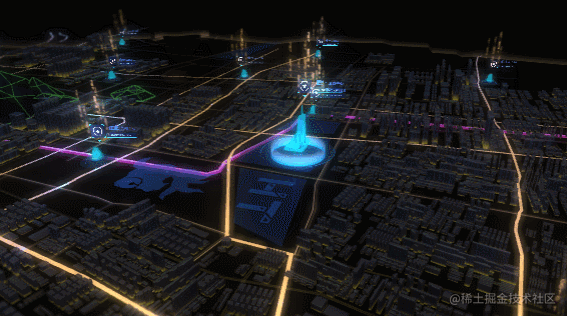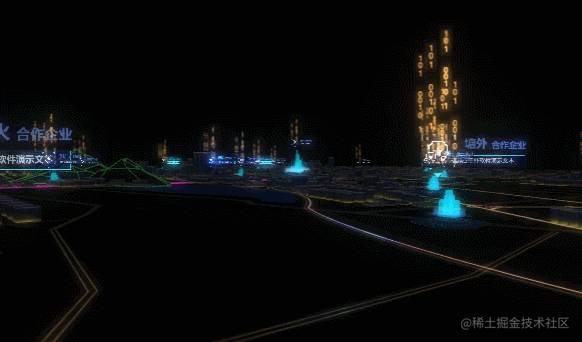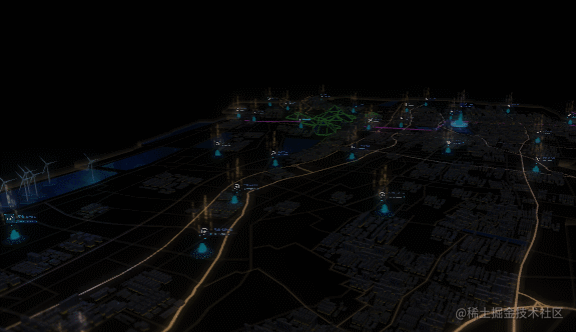onPostDeserialize(json, dm, view) {
const g3d = this.view;
g3d.setFar(100000);
const nodeUpArr1 = [], nodeUpArr2 = [], nodeUpArr3 = []；
//视角配置参数
const moveEyeConfig = [{
initEye:[-700,390,-974],
initCenter:[-1596,25,-518],
moveEye:[-2572, 390, -974],
moveCenter:[-1596,25,-518],
time: 9000,
eas: 'ease-in'
},{
initEye:[1500,71,900],
initCenter:[-1823,25,-636],
moveCenter:[-1823,25,-636],
moveEye:[-1678, 18, -558],
time:8000
},{
initEye:[2491,600,-1026],
initCenter:[0,0,0],
moveEye:[-3105, 500, -1577],
moveCenter:[-1034, -12, -41],
time:8000
}]
//创建一个蒙板div并返回cover函数
let cover = this.create2dCover();
//浮现城市的属性初始化
dm.each(fnode => {
//第一批楼房-市中心
if (fnode.getDisplayName() === "up1"){
fnode.a('startE',fnode.getElevation());
fnode.setElevation(-200);
nodeUpArr1.push(fnode);
}
//第二批城市-市中心附近建筑
if (fnode.getDisplayName() === "up2"){
fnode.a('startE',fnode.getElevation())
fnode.setElevation(-100);
nodeUpArr2.push(fnode);
}
//第三批城市-外围建筑
if (fnode.getDisplayName() === "up3"){
fnode.a('startE',fnode.getElevation())
fnode.setElevation(-100);
nodeUpArr3.push(fnode);
}

if(fnode.getDisplayName() === '飞光组'){
fnode.eachChild(node => {
node.s('shape3d.opacity',0);
})
}
54})

//视角开始变换
util.moveEyeAction(g3d,moveEyeConfig,cover)
//城市浮现
let upCityDemo = function(nodeArr,time,T = 0.6){
return new Promise((res,rej)=>{
ht.Default.startAnim({
duration:time,
action: (v,t) => {
nodeArr.forEach((node)=>{
if(t > T) res('已完成');
let org = node.getElevation();
let tar = node.a('startE');
node.setElevation(org + (tar - org) * v)
})
}
})
})
}

upCityDemo(nodeUpArr1,11000,0.4).then((res)=>{
// console.log(res)
return upCityDemo(nodeUpArr2,2000,0.4)
}).then((res)=>{
return upCityDemo(nodeUpArr3,2000);
}).then((res)=>{
//城市出现，开始动画
//this.startAnimation(g3d,dm);
})
84}复制代码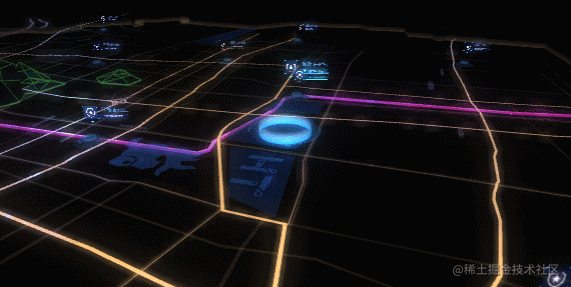· 建筑下的水波扩散动画

· 风车，建筑底下光圈旋转动画

· 道路偏移动画

· 市中心上方光线流动动画

· 建筑上面的数字飞光动画

ht.Default.startAnim({
frames: Infinity,
interval: 20,
action: () => {
//扩散水波动画
waveScale(scaleList,dltScale,maxScale,minScale);
//风车旋转，建筑底下光圈旋转
rotationAction(roationFC,dltRoattion);
rotationAction(roationD,dltRoattionD);
rotationAction(roationD2,-dltRoattionD2);
//道路偏移
//光亮建筑下的数字飞光
numberArr.forEach((node,index)=>{
})
//市中心上方亮线的流动
float.eachChild(node => {
let offset = node.s('shape3d.uv.offset') || [0, 0];
node.s('shape3d.uv.offset', [offset + 0.05, offset]);
})
}
});复制代码

//道路偏移动画
//定义三种道路的步进
//获取三种道路节点
let float = dm.getDataByTag('float');
//定义偏移动画函数
let uvFlow = function(obj,dlt){
let offset = obj.s('all.uv.offset') || [0, 0];
obj.s('all.uv.offset', [offset + dlt, offset]);
}

//水波缩放动画
//定义扩大范围和每步扩大速度
const maxScale = 1.5, dltScale = 0.06;
//获取缩放节点
let scaleList = dm.getDataByTag('scale');
//定义缩放函数
let waveScale = function(obj, dlt, max, min){
obj.eachChild(node => {
// 扩散半径增加
if (!node.a('max')) node.a('max', node.getScaleX() + max);
if (!node.s('shape3d.opacity')) node.s('shape3d.opacity',1);
let s = node.getScaleX() + dlt;
let y = node.getScale3d()
let opa = node.s('shape3d.opacity') - 0.02;
// 扩散半径大于最大值的时候，重置为最小值，透明度设为1
if (s >= node.a('max')){
opa = 1;
s = 0;
}
// 设置x,y,z方向的缩放值
node.s('shape3d.opacity',opa)
node.setScale3d(s, y, s);
});
}
//旋转图元
//定义三种不同旋转图元数组和旋转速度
const roationFC = [], roationD = [], roationD2 = [], dltRoattionD = Math.PI / 90, dltRoattionD2 = Math.PI / 60, dltRoattion = Math.PI / 30;
//获取所有旋转图元并分别放入数组中
let roationFCDatas = dm.getDataByTag('roationFC');
let roationdDatas = dm.getDataByTag('di');
roationFCDatas.eachChild(node =>{
node.eachChild(node => {
if (node.getDisplayName() === '风机叶片'){
roationFC.push(node);
}
})
});
roationdDatas.eachChild(node => {
if (node.getDisplayName() === '底'){
roationD.push(node)
}
if (node.getDisplayName() === '底2'){
roationD2.push(node)
}
});
//定义旋转函数
let rotationAction = function(obj,dlt){
obj.forEach(node => {
if (node.getDisplayName() === '风机叶片'){
//获得当前旋转角度
let rotationZ = node.getRotation3d();
//每步增加dlt
node.setRotation3d([0,0,rotationZ + dlt]);
}
if (node.getDisplayName() === '底' || node.getDisplayName() === '底2'){
//获得当前旋转角度
let rotationY = node.getRotation3d();
//每步增加dlt
node.setRotation3d([0,rotationY + dlt,0]);
}
})
}复制代码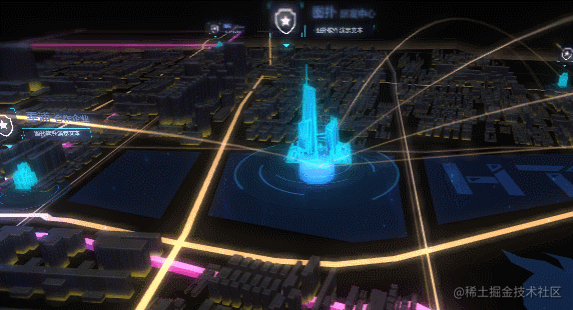//数字浮动
let numberArr, numFloadDis = 15, numFloatDlt = 0.07;
numberArr = new Array(28);
for (let i = 0;i < 28; i++){
numberArr[i] = new Array(6)
}
//产生两个随机数，并以数组形式返回
let randerdom2 = function(){
let num1 = Math.floor(Math.random() * 3);
let num2 = Math.floor((Math.random() * 3 + 3));
return [num1,num2];
}
//将所有的浮动数字按城市分组添加进数组
let i = 0,j=0;
dm.each(node => {
if (node.getDisplayName() === '飞光组'){
node.eachChild(node => {
node.s('shape3d.opacity',0);
node.setElevation(0);
numberArr[i][j++] = node;
})
j=0;
i++;
}
});
//属性初始化
let initArrAtr = function(){
for (let i = 0; i < numberArr.length; i++){
for (let j = 0; j < numberArr[i].length; j++){
//每条数字的随机数度
numberArr[i][j].a('randomSpeed', (numFloatDlt * 100 + Math.floor(Math.random() * 5))/100);
//控制每条数字是否停止上升
numberArr[i][j].a('stop',false);
//每栋楼上的已升起的飞光数量
numberArr[i].comNum = 0;
//每栋楼层当前的两条飞光
numberArr[i].one = randerdom2();
numberArr[i].two = randerdom2();
}
}
}
initArrAtr();
//重置单楼属性
let czArr = function(singleRoom){
//每栋楼上的已升起的数量
singleRoom.comNum = 0;
//重新随机设置每栋楼层出现的两条飞光
singleRoom.one = randerdom2();
singleRoom.two = randerdom2();
//设置飞光的随机速度
singleRoom.forEach((node, index)=>{
node.a('stop',false);
node.a('randomSpeed', (numFloatDlt * 100 + Math.floor(Math.random() * 5))/100);
})
}复制代码

let blockFloat = function(obj, dis){
//获取当前建筑
let allNumArr = obj;
//获取当前建筑出现的两条飞光
let floatArr = [allNumArr[allNumArr.one],allNumArr[allNumArr.two]];
let lth = floatArr.length;
//遍历并控制这两条飞光及动画
for (let j = 0; j < lth; j++){
let node = floatArr[j];
//如果当前飞光已停则停止此条飞光下一步动画
if (node.a('stop')) continue;
//获得当前飞光初始高度如果没有则手动设置当前为初始高度
let startE = node.a('startE');
if (startE == null) node.a('startE', startE = node.getElevation());
// 获得当前飞光速度和透明度值
let dlt = node.a('randomSpeed');
let float = node.a('float') || 0;
let opa = node.s('shape3d.opacity') || 0,

node.setElevation(startE + dis * float);
//上升的高度到达一定值设置透明度为1
if (float > 8){
node.s('shape3d.opacity',1)
}
//上升的高度到达最高则让当前建筑飞光到达数量加一，并停止进一步上升
if (float > 12){
allNumArr.comNum ++;
node.a('stop',true);
node.a('float', 0);
node.setElevation(startE);
node.s('shape3d.opacity',0);
//当前建筑飞光到达数量到达两条，重置建筑上所有飞光属性
if (allNumArr.comNum === 2){
czArr(allNumArr);
}
continue;
}
float += dlt;
node.s('shape3d.opacity',opa)
node.a('float', float);
}
}复制代码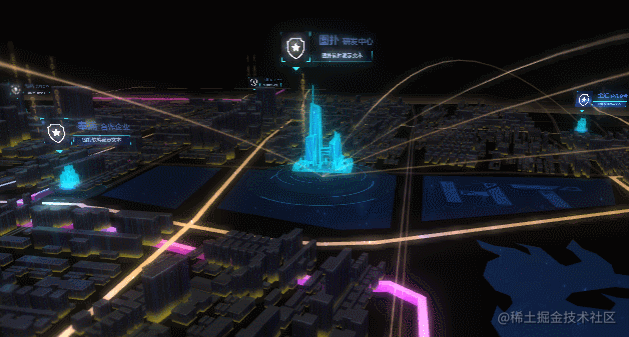（ps: 不仅如此，HT官网中 还包含了数百个工业互联网 2D 3D 可视化应用案例，点击这里体验把玩：www.hightopo.com/demos/index…)